# 基于OpenCV制作道路车辆计数应用程序

## 发展前景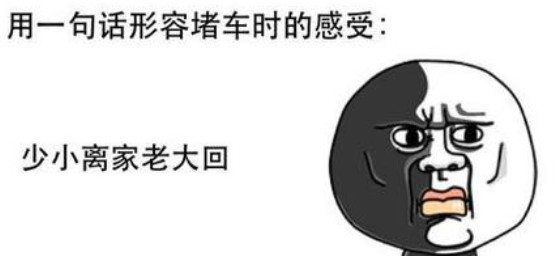1. 理解用于前景检测的背景减法算法的主要思想。

2. OpenCV图像过滤器。

3. 通过轮廓检测物体。

4. 构建处理管道以进一步处理数据。

# 背景减法算法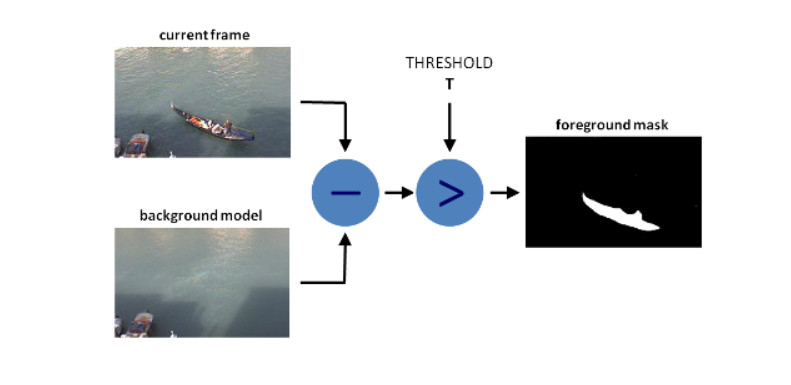foreground_objects = current_frame - background_layer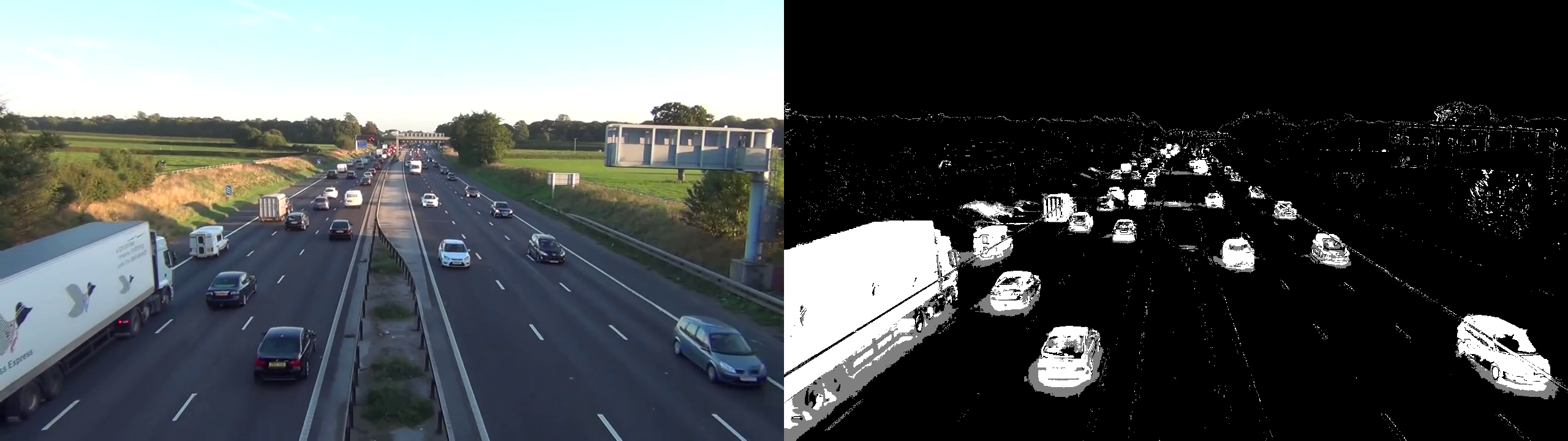def train_bg_subtractor(inst, cap, num=500):
'''
BG减法器需要处理一些帧来开始给出结果
'''
print('Training BG Subtractor...')
i = 0
while cap.isOpened():
inst.apply(frame, None, 0.001)
i += 1
if i >= num:
return cap
# 创造MOG BG减法器,缓存500帧和阴影剔除
# 设置图像源
cap = cv2.VideoCapture('input.mp4')
# 跳过500帧训练bg减法器
train_bg_subtractor(bg_subtractor, cap, num=500)# 过滤

#### 腐蚀

erosion = cv2.erode(img,kernel,iterations = 1)

#### 膨胀

dilation = cv2.dilate(img,kernel,iterations = 1)

#### 开运算（去噪）

opening = cv2.morphologyEx(img, cv2.MORPH_OPEN, kernel)

#### 闭运算（填黑点）

closing = cv2.morphologyEx(img, cv2.MORPH_CLOSE, kernel)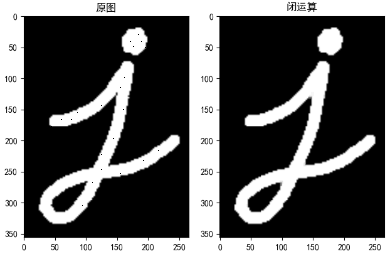def filter_mask(self, img, a=None):
# 这个过滤器是根据视觉测试手工挑选的
kernel = cv2.getStructuringElement(cv2.MORPH_ELLIPSE, (2, 2))
​
# 填补任何小洞
closing = cv2.morphologyEx(img, cv2.MORPH_CLOSE, kernel)
# 去除噪音
opening = cv2.morphologyEx(closing, cv2.MORPH_OPEN, kernel)
​
# 膨胀以合并相邻的小块
dilation = cv2.dilate(opening, kernel, iterations=2)
​
return dilation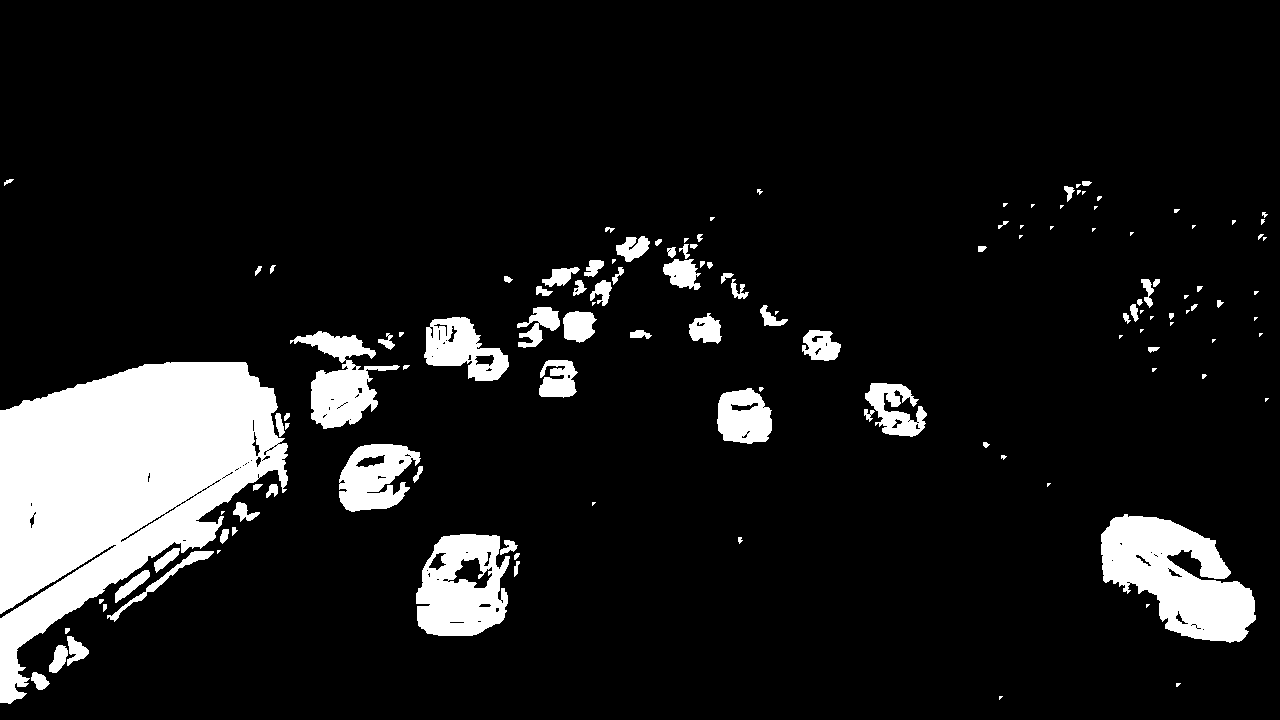# 通过轮廓检测物体

cv2.CV_RETR_EXTERNAL  - 仅获取外轮廓。cv2.CV_CHAIN_APPROX_TC89_L1  - 使用Teh-Chin链式近似算法（更快）
# 得到边界框的中心
def get_centroid(x, y, w, h):
x1 = int(w / 2)
y1 = int(h / 2)

cx = x + x1
cy = y + y1

return (cx, cy)

matches = []
# 发现外部轮廓
contours, hierarchy = cv2.findContours(

# 根据高度，宽度进行过滤
for (i, contour) in enumerate(contours):
# 找到其中一条轮廓,进行高和宽的过滤
(x, y, w, h) = cv2.boundingRect(contour)
contour_valid = (w >= min_contour_width) and (
h >= min_contour_height)

if not contour_valid:
# 不满足过滤掉
continue

# 得到边界框的中心
centroid = get_centroid(x, y, w, h)
# 将轮廓加入数组中
matches.append(((x, y, w, h), centroid))

return matches

# Pipeline封装

class PipelineRunner(object):
'''
非常简单的pipline。
只需按顺序运行处理程序，将上下文从一个传递到另一个。
您还可以为处理器设置日志级别。
'''
​
def __init__(self, pipeline=None, log_level=logging.DEBUG):
self.pipeline = pipeline or []
self.context = {}
self.log = logging.getLogger(self.__class__.__name__)
self.log.setLevel(log_level)
self.log_level = log_level
self.set_log_level()
​
def set_context(self, data):
self.context = data
​
if not isinstance(processor, PipelineProcessor):
raise Exception(
'Processor should be an isinstance of PipelineProcessor.')
processor.log.setLevel(self.log_level)
self.pipeline.append(processor)
​
def remove(self, name):
for i, p in enumerate(self.pipeline):
if p.__class__.__name__ == name:
del self.pipeline[i]
return True
return False
​
def set_log_level(self):
for p in self.pipeline:
p.log.setLevel(self.log_level)
​
def run(self):
for p in self.pipeline:
self.context = p(self.context)
​
self.log.debug("Frame #%d processed.", self.context['frame_number'])
​
return self.context
​
​
class PipelineProcessor(object):
'''
处理器的基类。
'''
​
def __init__(self):
self.log = logging.getLogger(self.__class__.__name__)

class ContourDetection(PipelineProcessor):
'''
检测移动物体。
这个处理器的目的是减去背景，获得移动物体
并使用cv2.findContours方法检测它们，然后过滤掉宽度和高度不符合的。
bg_subtractor  - 背景减法器isinstance。
min_contour_width  - 最小边界矩形宽度。
min_contour_height  - 最小边界矩形高度。
save_image  - 如果为True，则将检测到的对象掩码保存到文件中。
image_dir  - 保存图像的位置（必须存在）。
'''
​
def __init__(self, bg_subtractor, min_contour_width=35, min_contour_height=35, save_image=False,
image_dir='images'):
super(ContourDetection, self).__init__()
​
self.bg_subtractor = bg_subtractor
self.min_contour_width = min_contour_width
self.min_contour_height = min_contour_height
self.save_image = save_image
self.image_dir = image_dir
​
# 这个过滤器是根据视觉测试手工挑选的
kernel = cv2.getStructuringElement(cv2.MORPH_ELLIPSE, (2, 2))
​
# 闭运算，填补任何小洞
closing = cv2.morphologyEx(img, cv2.MORPH_CLOSE, kernel)
# 开运算，去除噪音
opening = cv2.morphologyEx(closing, cv2.MORPH_OPEN, kernel)
​
# 膨胀以合并相邻的小块
dilation = cv2.dilate(opening, kernel, iterations=2)
​
return dilation
​
​
matches = []
​
# 发现外部轮廓
contours, hierarchy = cv2.findContours(fg_mask, cv2.RETR_EXTERNAL, cv2.CHAIN_APPROX_TC89_L1)
for (i, contour) in enumerate(contours):
# 找到其中一条轮廓,进行高和宽的过滤
(x, y, w, h) = cv2.boundingRect(contour)
contour_valid = (w >= self.min_contour_width) and (
h >= self.min_contour_height)
​
if not contour_valid:
# 不满足过滤掉
continue
# 得到边界框的中心
centroid = utils.get_centroid(x, y, w, h)
# 将轮廓加入数组中
matches.append(((x, y, w, h), centroid))
​
return matches
​
def __call__(self, context):
frame = context['frame'].copy()
frame_number = context['frame_number']
​
# 只是阈值化
if self.save_image:
#可选,保存过滤后的黑白图片
#进行目标检测
​
return context

class VehicleCounter(PipelineProcessor):
'''
计算进入出口区域的车辆。
此类的目的是基于检测到的对象和本地缓存创建
对象修补和计数在退出区域中定义的退出区域中输入的对象。
path_size  - 路径中的最大点数。
max_dst  - 两点之间的最大距离。
'''
​
def __init__(self, exit_masks=[], path_size=10, max_dst=30, x_weight=1.0, y_weight=1.0):
super(VehicleCounter, self).__init__()
​
​
self.vehicle_count = 0
self.path_size = path_size
self.pathes = []
self.max_dst = max_dst
self.x_weight = x_weight
self.y_weight = y_weight
​
def check_exit(self, point):
try:
return True
except:
return True
return False
​
def __call__(self, context):
objects = context['objects']
context['pathes'] = self.pathes
context['vehicle_count'] = self.vehicle_count
if not objects:
return context
​
points = np.array(objects)[:, 0:2]
points = points.tolist()
​
# 如果pathes为空，则添加新点
if not self.pathes:
for match in points:
self.pathes.append([match])
​
else:
# 根据最小距离链接新点与旧路径
# points
new_pathes = []
​
for path in self.pathes:
_min = 999999
_match = None
for p in points:
if len(path) == 1:
# distance from last point to current
d = utils.distance(p, path[-1])
else:
# 基于前2个点预测下一个点并计算
# 从预测的下一个点到当前的距离
xn = 2 * path[-1] - path[-2]
yn = 2 * path[-1] - path[-2]
d = utils.distance(
p, (xn, yn),
x_weight=self.x_weight,
y_weight=self.y_weight
)
​
if d < _min:
_min = d
_match = p
​
if _match and _min <= self.max_dst:
points.remove(_match)
path.append(_match)
new_pathes.append(path)
​
# 如果当前帧没有匹配，则不丢弃路径
if _match is None:
new_pathes.append(path)
​
self.pathes = new_pathes
​
# 添加新的pathes
if len(points):
for p in points:
# 不添加已经计算的点数
if self.check_exit(p):
continue
self.pathes.append([p])
​
# 仅保存路径中的最后N个点
for i, _ in enumerate(self.pathes):
self.pathes[i] = self.pathes[i][self.path_size * -1:]
​
# 计算车辆和去除计数pathes：
new_pathes = []
for i, path in enumerate(self.pathes):
d = path[-2:]
​
if (
# 需要列表中的两点来计算
len(d) >= 2 and
# 上一个点不是在出口区
not self.check_exit(d) and
# 当前点在出口区
self.check_exit(d) and
# path len 大于 path_size
self.path_size <= len(path)
):
self.vehicle_count += 1
else:
# 禁止链接已经在退出区域中的路径
for p in path:
if self.check_exit(p):
break
new_pathes.append(path)
​
self.pathes = new_pathes
​
context['pathes'] = self.pathes
context['objects'] = objects
context['vehicle_count'] = self.vehicle_count
​
self.log.debug('#VEHICLES FOUND: %s' % self.vehicle_count)
​
return context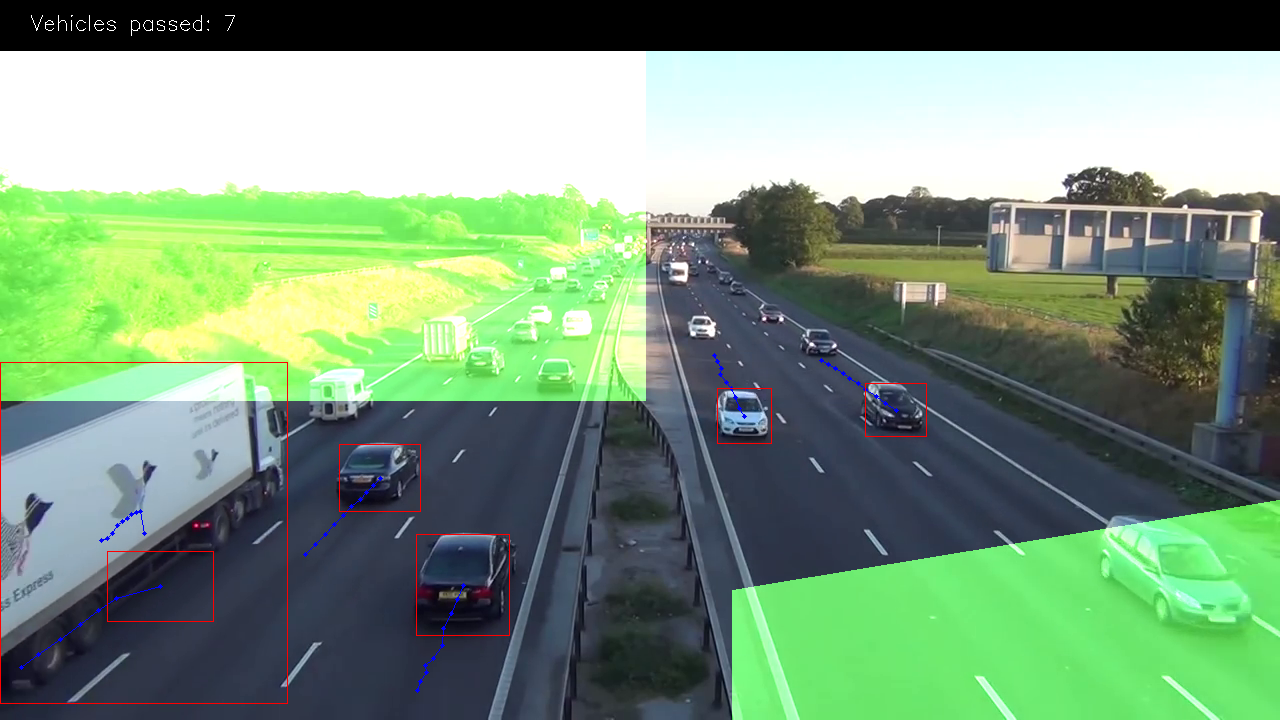EXIT_PTS = np.array([
[[732, 720], [732, 590], [1280, 500], [1280, 720]],
[[0, 400], [645, 400], [645, 0], [0, 0]]
])

base = np.zeros(SHAPE + (3,), dtype='uint8')
exit_mask = cv2.fillPoly(base, EXIT_PTS, (255, 255, 255))[:, :, 0]

# 根据最小距离链接新点与旧路径
# points
new_pathes = []
​
for path in self.pathes:
_min = 999999
_match = None
for p in points:
if len(path) == 1:
# distance from last point to current
d = utils.distance(p, path[-1])
else:
# 基于前2个点预测下一个点并计算
# 从预测的下一个点到当前的距离
xn = 2 * path[-1] - path[-2]
yn = 2 * path[-1] - path[-2]
d = utils.distance(
p, (xn, yn),
x_weight=self.x_weight,
y_weight=self.y_weight
)
​
if d < _min:
_min = d
_match = p
​
if _match and _min <= self.max_dst:
points.remove(_match)
path.append(_match)
new_pathes.append(path)
​
# 如果当前帧没有匹配，则不丢弃路径
if _match is None:
new_pathes.append(path)
​
self.pathes = new_pathes
​
# 添加新的pathes
if len(points):
for p in points:
# 不添加已经计算的点数
if self.check_exit(p):
continue
self.pathes.append([p])
​
# 仅保存路径中的最后N个点
for i, _ in enumerate(self.pathes):
self.pathes[i] = self.pathes[i][self.path_size * -1:]

# 计算车辆和去除计数pathes：
new_pathes = []
for i, path in enumerate(self.pathes):
d = path[-2:]
​
if (
# 需要列表中的两点来计算
len(d) >= 2 and
# 上一个点不是在出口区
not self.check_exit(d) and
# 当前点在出口区
self.check_exit(d) and
# path len 大于 path_size
self.path_size <= len(path)
):
self.vehicle_count += 1
else:
# 禁止链接已经在退出区域中的路径
for p in path:
if self.check_exit(p):
break
new_pathes.append(path)
​
self.pathes = new_pathes
​
context['pathes'] = self.pathes
context['objects'] = objects
context['vehicle_count'] = self.vehicle_count
​
self.log.debug('#VEHICLES FOUND: %s' % self.vehicle_count)

class Visualizer(PipelineProcessor):
​
def __init__(self, save_image=True, image_dir='images'):
super(Visualizer, self).__init__()
​
self.save_image = save_image
self.image_dir = image_dir
​
return True
return False
# 绘制路径
def draw_pathes(self, img, pathes):
if not img.any():
return
​
for i, path in enumerate(pathes):
path = np.array(path)[:, 1].tolist()
for point in path:
# 画出圆点和蓝色线条实现车尾尾随效果
cv2.circle(img, point, 2, CAR_COLOURS, -1)
cv2.polylines(img, [np.int32(path)], False, CAR_COLOURS, 1)
​
return img
# 绘制包裹车的盒子
for (i, match) in enumerate(pathes):
​
contour, centroid = match[-1][:2]
continue
​
x, y, w, h = contour
# 根据车的宽高，绘制出车的矩形大小
cv2.rectangle(img, (x, y), (x + w - 1, y + h - 1),
BOUNDING_BOX_COLOUR, 1)
cv2.circle(img, centroid, 2, CENTROID_COLOUR, -1)
​
return img
​
​
# 这只是为图像添加不透明度的绿色遮罩
_img = np.zeros(img.shape, img.dtype)
_img[:, :] = EXIT_COLOR
# 在图片上面绘制计数结果
cv2.rectangle(img, (0, 0), (img.shape, 50), (0, 0, 0), cv2.FILLED)
cv2.putText(img, ("Vehicles passed: {total} ".format(total=Zvehicle_count)), (30, 30),
cv2.FONT_HERSHEY_SIMPLEX, 0.7, (255, 255, 255), 1)
return img
​
def __call__(self, context):
frame = context['frame'].copy()
frame_number = context['frame_number']
pathes = context['pathes']
vehicle_count = context['vehicle_count']
​
frame = self.draw_pathes(frame, pathes)
​
utils.save_frame(frame, self.image_dir +
"/processed_%04d.png" % frame_number)
​
return context

CSV编写器正在按时间保存数据，因为我们需要它来进行进一步的分析。所以我使用这个公式为unixtimestamp添加额外的帧时序：

time =（（self.start_time + int（frame_number / self.fps））* 100+ int（100.0 / self.fps）*（frame_number％self.fps））

# 结论

## 附加：

### yolo 算法

v1是yolo系列的开山之作，以简洁的网络结构，简单的复现过程而受到人们的追捧。 yolo_v1奠定了yolo系列算法“分而治之”的基调，在yolo_v1上，输入图片被划分为7X7的网格，如下图所示: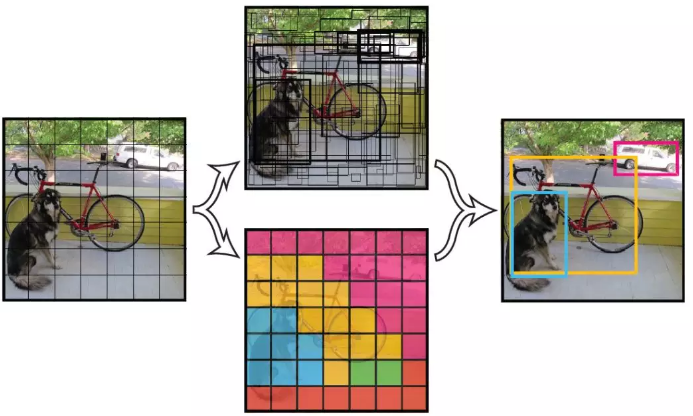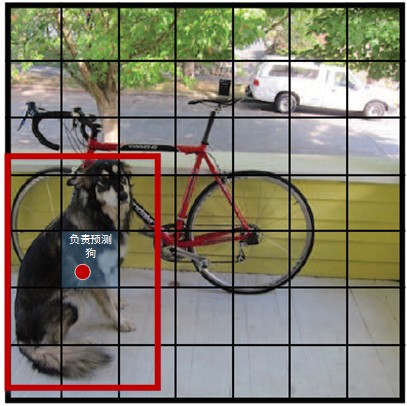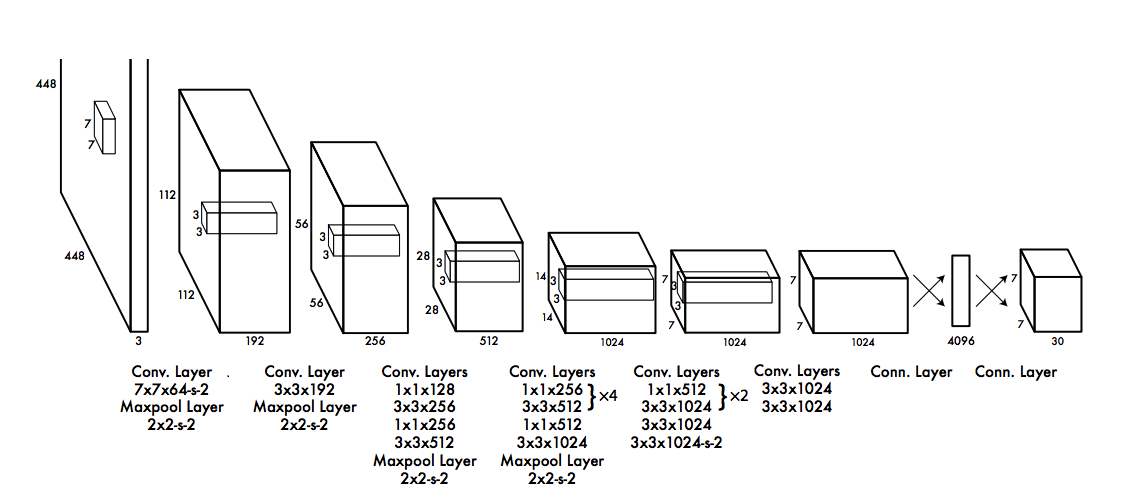1. 使用了leaky ReLU，相比普通ReLU，leaky并不会让负数直接为0

2. 分而治之，用网格来划分图片区域，每块区域独立检测目标；

3. 端到端训练。损失函数的反向传播可以贯穿整个网络，这也是one-stage检测算法的优势。

YOLO局限性：

#### 效果：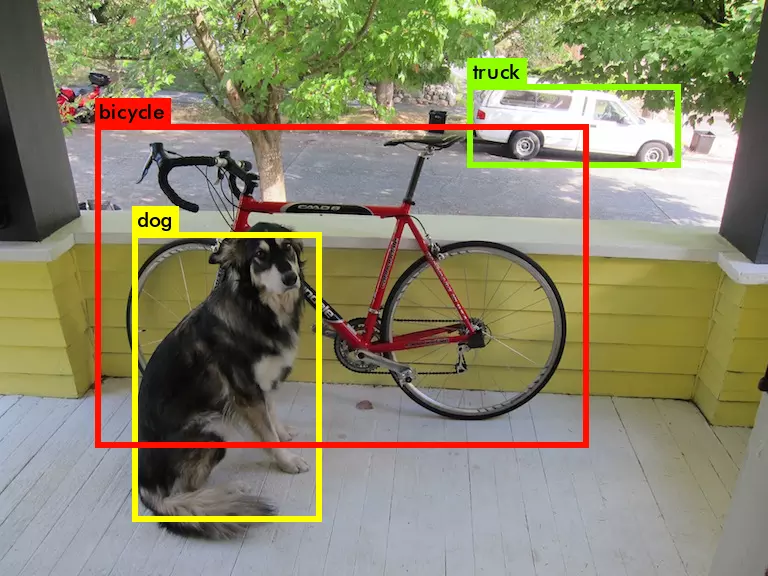posted @ 2019-08-27 11:00  Timcode  阅读(1162)  评论(0编辑  收藏